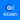# Solution: What is the derivative with respect to x of 2cos^2⁡(x^2+2)?

(Last Updated On: January 22, 2020)#### Problem Statement: CE Board November 1995

What is the derivative with respect to x of 2cos^2⁡(x^2+2)?

• A. 2sin (x^2 + 2) cos (x^2 + 2)
• B. -2sin (x^2 + 2) cos (x^2 + 2)
• C. 8x sin (x^2 + 2) cos (x^2 + 2)
• D. -8x sin (x^2 + 2) cos (x^2 + 2)

The derivative is equal to 8xsin(x^2 + 2)cos(x^2 + 2)

#### Latest Problem Solving in Differential Calculus (LIMITS & DERIVATIVES)

More Questions in: Differential Calculus (LIMITS & DERIVATIVES)

#### Online Questions and Answers in Differential Calculus (LIMITS & DERIVATIVES)

Help Me Makes a Difference!

 P inoyBIX educates thousands of reviewers/students a day in preparation for their board examinations. Also provides professionals with materials for their lectures and practice exams. Help me go forward with the same spirit. “Will you make a small gift today via GCASH?”+63 966 459 6474 Option 1 : \$1 USD Option 2 : \$3 USD Option 3 : \$5 USD Option 4 : \$10 USD Option 5 : \$25 USD Option 6 : \$50 USD Option 7 : \$100 USD Option 8 : Other Amount© 2014 PinoyBIX Engineering. © 2019 All Rights Reserved | How to Donate? |